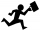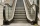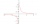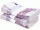# Fraction + eq

Solve following simple equation with fractions:

-5/6(8+5b) = 75 + 5/3b

Result

b =  -14

#### Solution:

-5/6(8+5b) = 75 + 5/3b

35b = -490

b = -14

Calculated by our simple equation calculator.

Leave us a comment of this math problem and its solution (i.e. if it is still somewhat unclear...):Math student
2/6 divided by 2/4Dr Math#### Following knowledge from mathematics are needed to solve this word math problem:

Need help calculate sum, simplify or multiply fractions? Try our fraction calculator. Do you have a linear equation or system of equations and looking for its solution? Or do you have quadratic equation?

## Next similar math problems:

1. Two workersOne worker needs 40 hours to do a job, and the second would do it in 30 hours. They worked together for several hours, then the second was recalled, and the first completed the job itself in 5 hours. How many hours did they work together, and how much did.
2. Discount and pricesAfter a 29% discount, the new product price was € 426. What was the original price of the product?
3. Faces diagonalsIf the diagonals of a cuboid are x, y, and z (wall diagonals or three faces) respectively than find the volume of a cuboid. Solve for x=1.2, y=1.7, z=1.45
4. Simple equation 1035= 7*3*x what is x?
5. Secret treasureScouts have a tent in the shape of a regular quadrilateral pyramid with a side of the base 4 m and a height of 3 m. Determine the radius r (and height h) of the container so that they can hide the largest possible treasure.
6. The hemisphereThe hemisphere container is filled with water. What is the radius of the container when 10 liters of water pour from it when tilted 30 degrees?
7. Floating of wood - Archimedes lawWhat will be the volume of the floating part of a wooden (balsa) block with a density of 200 kg/m3 and a volume of 0.02 m3 that floats in alcohol? (alcohol density is 789 kg/m3)
8. Sides of right angled triangleOne leg is 1 m shorter than the hypotenuse, and the second leg is 2 m shorter than the hypotenuse. Find the lengths of all sides of the right-angled triangle.
9. Two chordsCalculate the length of chord AB and perpendicular chord BC to circle if AB is 4 cm from the center of the circle and BC 8 cm from the center of the circle.
10. Before yesterdayHe merchant adds a sale sign in his shop window to the showed pair of shoes in the morning: "Today by p% cheaper than yesterday. " After a while, however, he decided that the sign saying: "Today 62.5% cheaper than the day before yesterday". Determine the n
11. AM of three numbersThe number 2010 can be written as the sum of 3 consecutive natural numbers. Determine the arithmetic mean of these numbers.
12. The escalatorI run up the escalator at a constant speed in the direction of the stairs and write down the number of steps A we climbed. Then we turn around and run it at the same constant speed in the opposite direction and write down the number of steps B that I climb
13. Equation 23Find value of unknown x in equation: x+3/x+1=5 (problem finding x)
14. Geography testsOn three 150-point geography tests, you earned grades of 88%, 94%, and 90%. The final test is worth 250 points. What percent do you need on the final to earn 93% of the total points on all tests?
15. Medians in right triangleIt is given a right triangle, angle C is 90 degrees. I know it medians t1 = 8 cm and median t2 = 12 cm. .. How to calculate the length of the sides?
16. Aunt RoseAunt Rose gave \$2500 to Mani and Cindy. Mani received \$500 more than Cindy. How mich did Cindy received?
17. 45th birthdayThis year Mrs. Clever celebrated her 45th birthday. Her three children are now 7.11 and 15 years old. In how many years will Mrs. Clever's age be equal to the sum of her children's years?